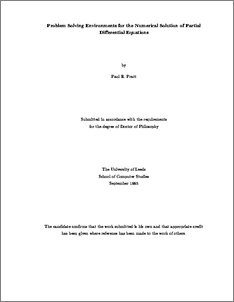# Problem solving environments for the numerical solution of partial differential equations

Pratt, P. (1996) Problem solving environments for the numerical solution of partial differential equations. PhD thesis, University of Leeds.Preview
Text
pratt.pdf